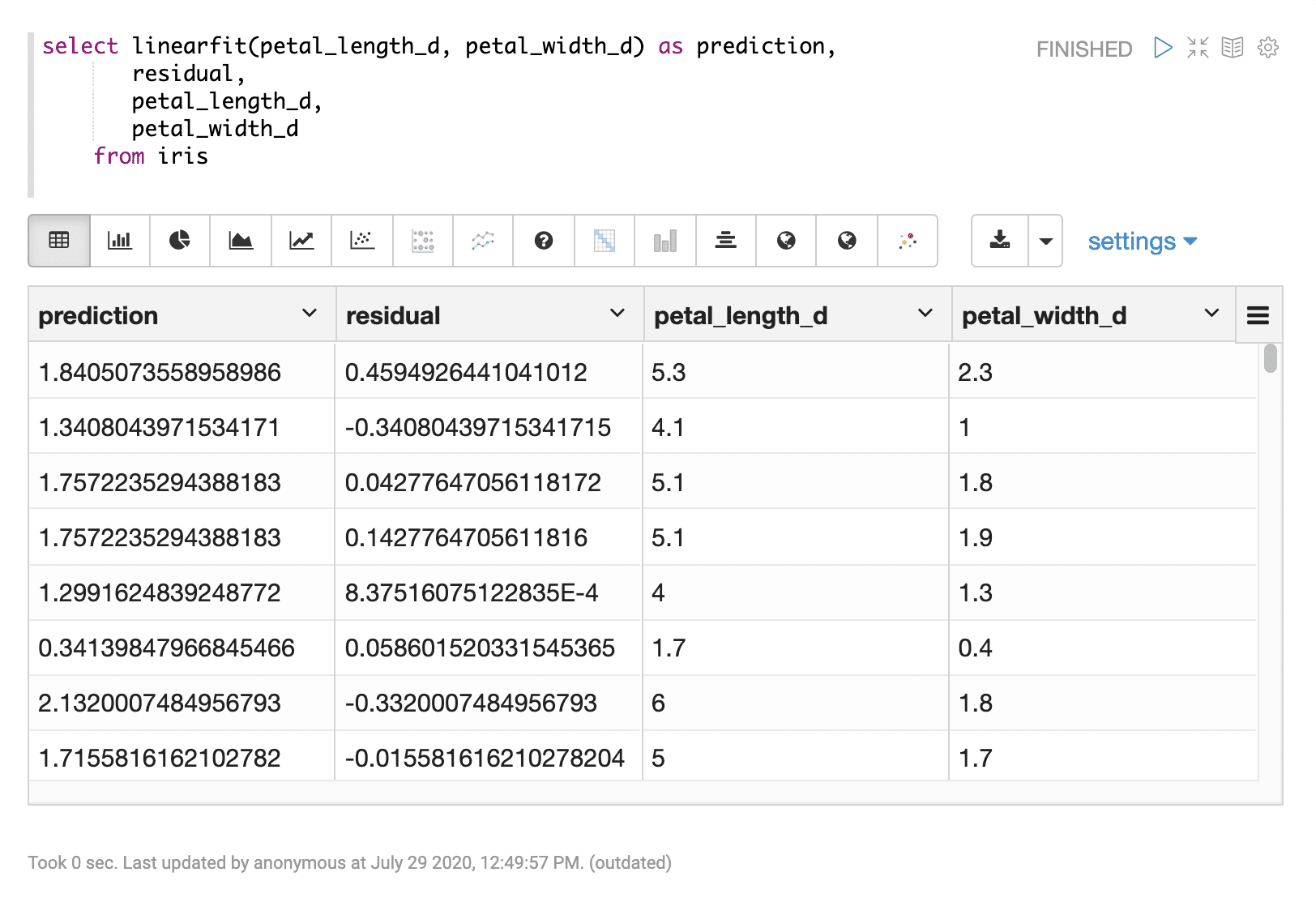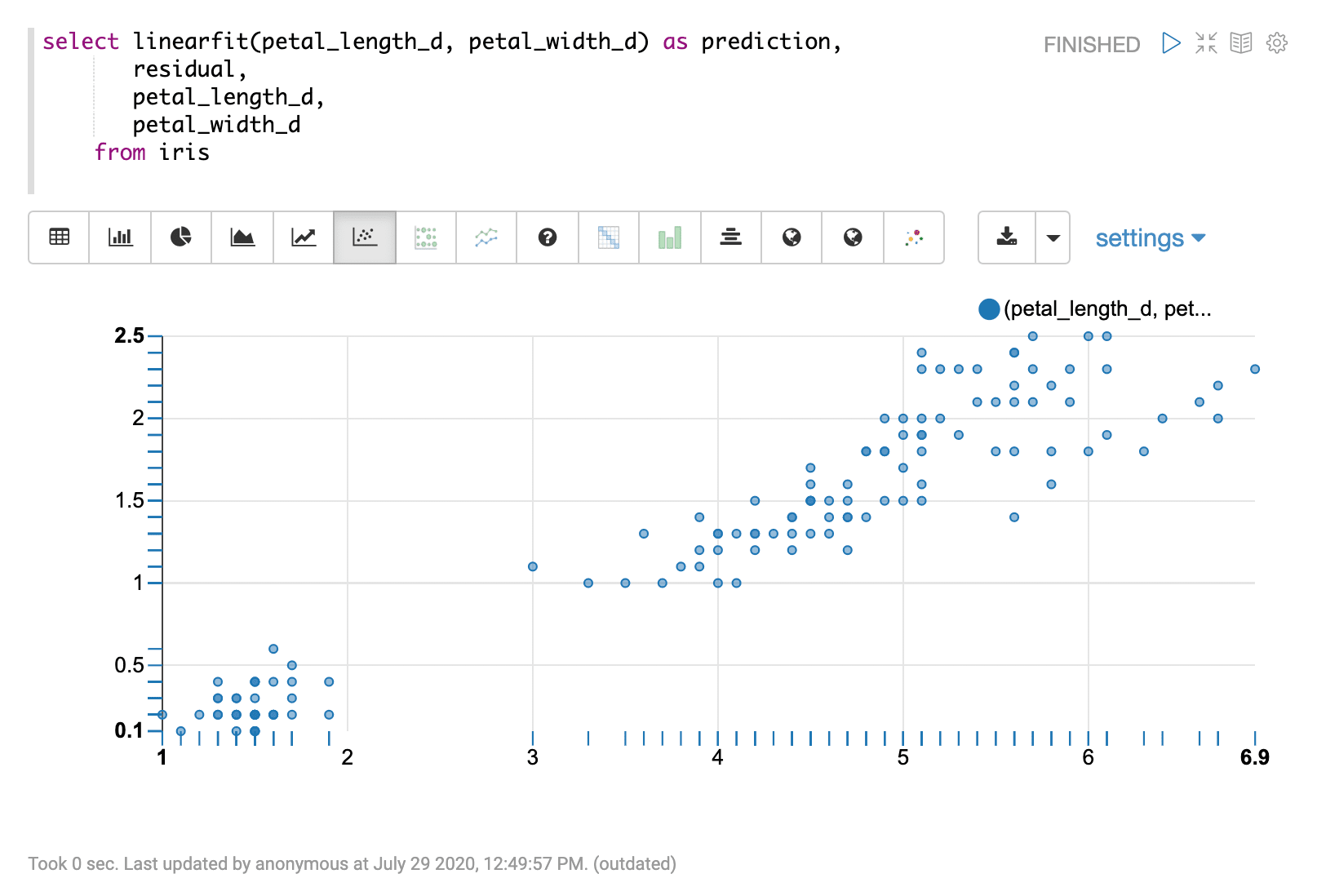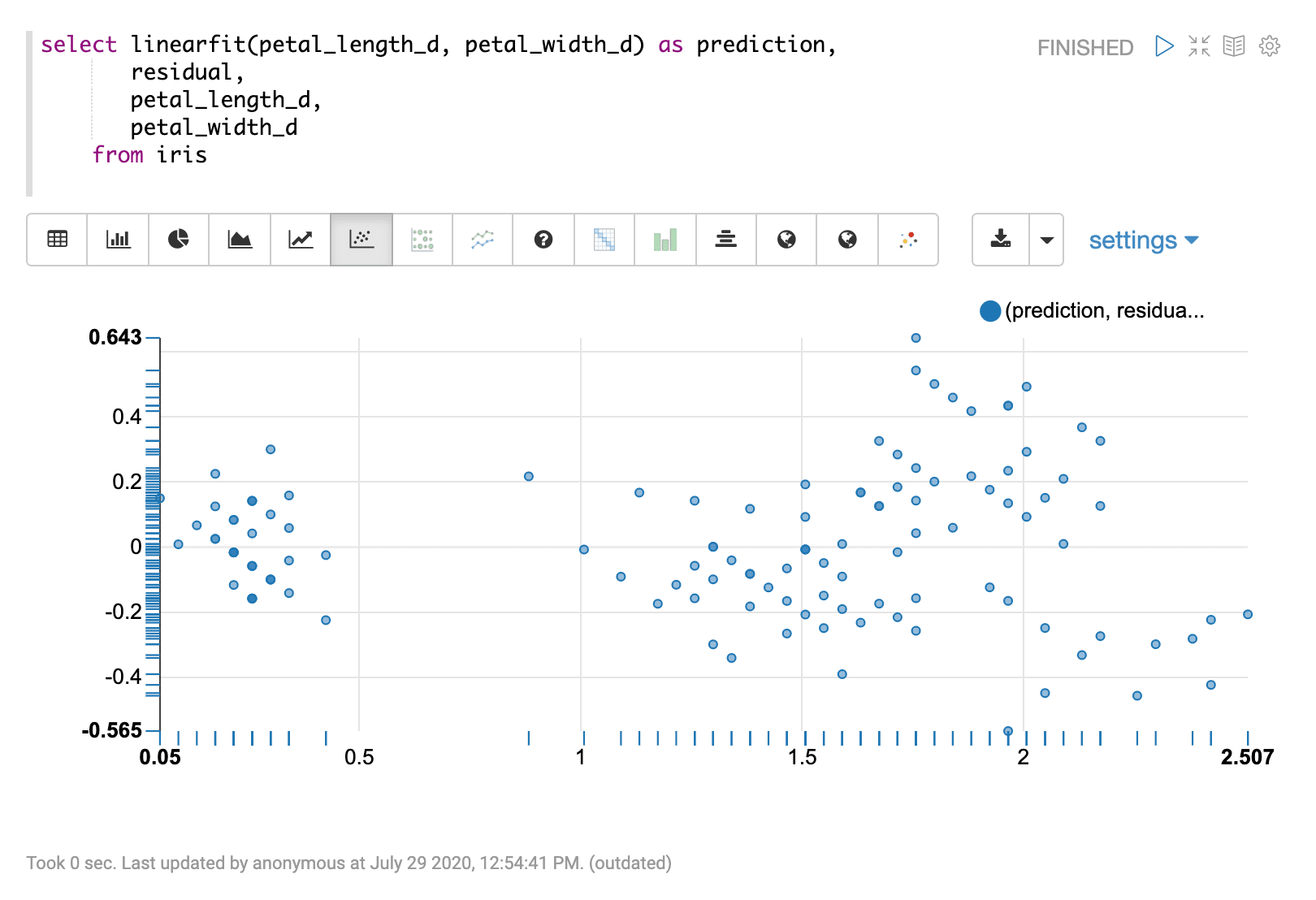# Regression and Residual Plot (linearfit)

The `linearfit` function uses linear regression to predict a straight line through a bi-variate scatter plot. The `linearfit` function takes two parameters:

1. The numeric field containing the independent (x) variable.

2. The numeric field containing the dependent (y) varlineiable.

## Sample syntax

``````select linearfit(petal_length_d, petal_width_d) as prediction,
residual,
petal_length_d,
petal_width_d
from iris
limit 150``````

## Result set

The result set contains a random sample of records that match the `WHERE` clause. If no `WHERE` clause is included the random sample will be taken from the entire result set. The size of the random sample can be controlled by the `LIMIT` clause. The default sample size, if no limit is applied, is 25,000.

The `linearfit` function returns the predicted value for each record. There are three additional fields that can be selected when the `linearfit` function is used:

• `residual`: The residual value for each sample. The residual value is the samples dependent (y) value minus the predicted value. The residual represents the error of the regression prediction for each sample.

• The independent variable for each sample.

• The dependent variable for each sample.

Sample result set shown in Apache Zeppelin## Visualizations

There are a number of visualizations that can flow from the regression result set.

The first visualization shown is a scatter plot with `petal_length_d` on the x-axis and `petal_width_d` on the y-axis. This can be used to visualize the relationship between the two variables in the regression analysis.The second visualization shows the `petal_length_d` variable on the x-axis and the prediction for `petal_width_d` on y-axis.The last visualization plots the predictions on the x-axis and the residual on the y-axis. This residual plot can be used to visualize the error of the regression model across the full range of predictions.